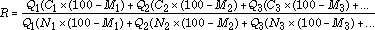# Calculate C/N Ratio For Three Materials

This calculation solves for the carbon to nitrogen ratio of up to three materials. Enter the mass of each material (wet weight), percentage of carbon, percentage of nitrogen, and percentage of moisture, then click on the calculate button. If you have less than three materials be sure to enter zeroes in the fields for the missing materials.

Note - Use whole numbers

Ingredient
% H20
Weight
% Carbon
% Nitrogen
C/N Ratio
Result:

Here's the Formula:
Given overall moisture percentage goal of (g)

Ingredient %H20 Weight %Carbon %Nitrogen C/N Ratio
1 M1 Q1 C1 N1
2 M2 Q2 C2 N2
3 M3 Q3 C3 N3
R```

in which:

R = C/N ratio

Qn = mass of material n ("as is", or "wet weight")

Cn = carbon (%)

Nn = nitrogen (%)

Mn = moisture content (%) of material n

```

Cornell Waste Management Institute © 1996
Dept of Crop and Soil Sciences